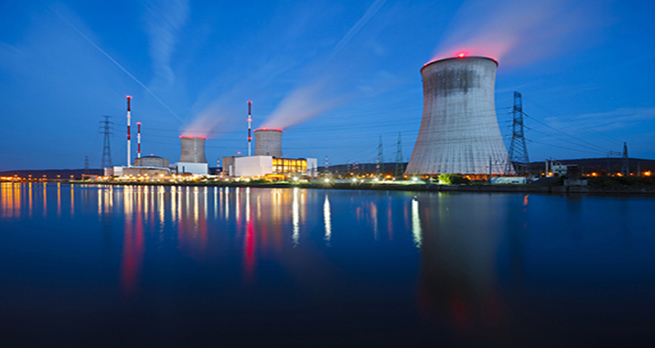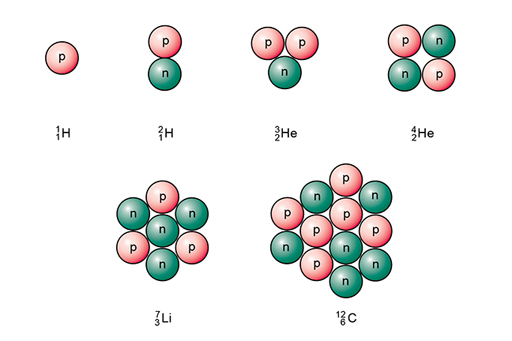Science, Maths & Technology

### Become an OU studentThe science of nuclear energy

Start this free course now. Just create an account and sign in. Enrol and complete the course for a free statement of participation or digital badge if available.

# 1.1.1 Isotopes

So, the properties of a given element are determined by the number of electrons and protons within its atoms.

The number of protons determines what the element is. For example, all atoms of carbon have six protons, and any atom containing six protons must be carbon.

## What role do the neutrons play?

An element can have different forms wherein the atoms have the same number of protons but a different number of neutrons. These different forms are called isotopes. The number of neutrons in an atom can be worked out from the mass number given the symbol A. The mass number is equal to the number of protons in a nucleus plus the number of neutrons.

So:

• Mass number = number of neutrons + number of protons
• A = number of neutrons + Z
• Number of neutrons = A−Z

The number of neutrons in an isotope can be found from the difference between the mass number and the atomic number.

As a shorthand, isotopes of each element may be represented by using the following notation . X is the symbol for the element itself and two numbers are used to indicate the atomic number (lower number, Z) and mass number (upper number, A).

So, a normal hydrogen atom is represented as and an atom of a heavier isotope, deuterium, as . Isotopes of some other light atoms are shown in Figure 3.Figure 3 A schematic diagram of the nuclei of some isotopes.

An alternative notation is to use the name of the element followed by a hyphen and then the mass number. For example, helium would usually be denoted by helium-4, but the lighter isotope referred to above would be given as helium-3. These can also be abbreviated to just the chemical symbol and mass number, for example, He-4 and He-3.

In the next section, you will discover more about isotopes.